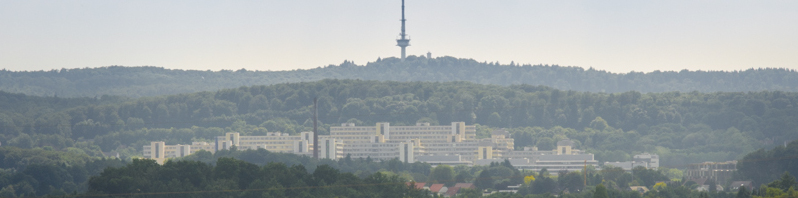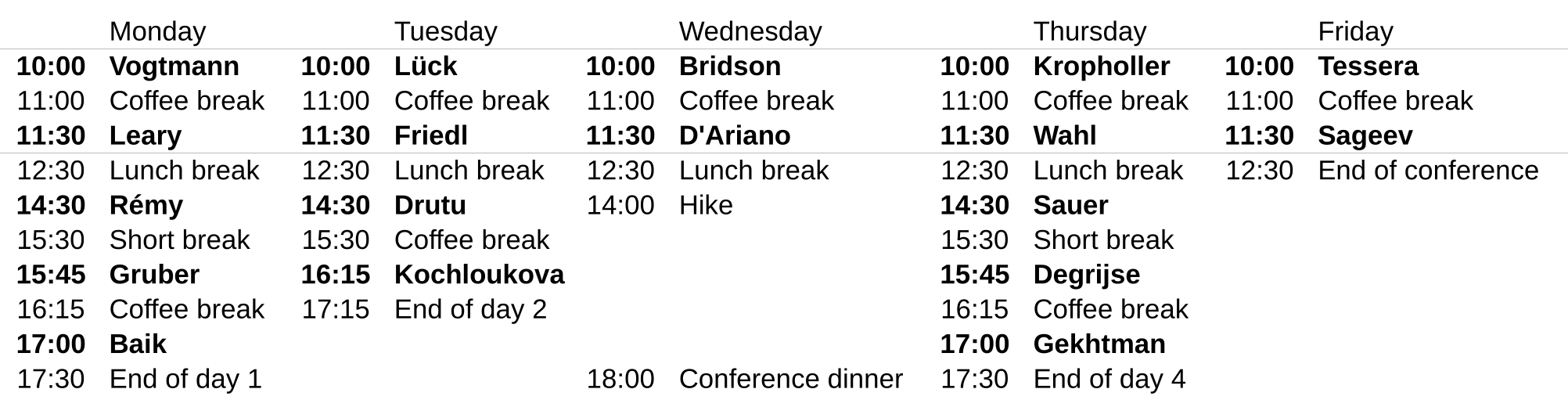Conference "Topological and Homological Methods in Group Theory"# Programme

## ScheduleThe schedule may still be subject to change

## Talks

All talks will be held in lecture hall H5, H6.

• A discrete group of circle homeomorphisms is a Fuchsian group if and only if it is a convergence group (this is due to Tukia, Casson-Jungreis, Gabai, ...). We show that the convergence property can also be characterized in terms of invariant laminations on the circle, so this gives a new characterization of Fuchsian groups. We also discuss what can be said about fibered hyperbolic 3-manifold groups. The main motivation of the work is Thurston´s universal circle theory.
• I'll present joint work with Dessislava Kochloukova (Campinas) in which we explore the asymptotic growth of homology groups and the cellular volume of classifying spaces as one passes to normal subgroups of increasing finite index in a residually finite group satisfying appropriate finiteness conditions. I shall also present results concerning the virtual first betti number of solvable groups.
• I will show how a recent axiomatic derivation of quantum theory and of quantum field theory leads to quantum walks on Cayley graphs. Although it has a thorough physical interpretation, the new axiomatization is purely mathematical, namely it has no physical primitives (i.e. it assumes no mechanics, no special relativity, no space-time). I will show how the usual free quantum field theory (Weyl, Maxwell, and Dirac) is recovered in the so-called "relativistic limit", briefly illustrating how the whole physics emerges, how the principle of relativity is formulated in mathematical terms, how for Dirac mass m=0 Lorentz symmetry is recovered in the relativistic limit, whereas a De Sitter symmetry is recovered for m>0. This fact, along with other features having general-relativistic flavor are promising for an alternative approach to quantum gravity. The construction poses to theoretical physicists problems of geometric group theory, a field that is out of the span of current mathematical physics, and is largely unknown to theoretical physicists.
• In this talk I will explain how to construct, for every positive integer $r$, an example of a word-hyperbolic group $G_r$ whose outer automorphism group $\mathrm{Out}(G_r)$ is virtually torsion-free and admits a cocompact classifying space for proper actions, but such that the minimal dimension of such a space is strictly larger than the virtual cohomological dimension of $\mathrm{Out}(G_r)$ plus $r$ . This is joint work with Juan Souto.
• Various strengthened versions of property (T) have been formulated in recent years. Among them, those involving actions on $L^p$ spaces are particularly interesting, because they manage to achieve a separation between rank one and higher rank lattices, because of their presumed connection to the conformal dimension of the boundary of hyperbolic groups, and because of the increasing role that they play in operator algebras.
In this talk I shall explain how random groups have all the strengthened $L^p$-versions of property (T). This is joint work with J. Mackay.
• We will assign a marked polytope to any two-generator one-relator group G. The polytope contains information on the BNS-invariants, and in case that G is the fundamental group of a 3-manifold M the polytope determines the Thurston norm of M. This talk is based on work with Wolfgang Lueck, Kevin Schreve and Stephan Tillmann.
• Consider any nonelementary action of a hyperbolic group G on a not necessarily proper Gromov hyperbolic space X. The action is not assumed to be discrete and X is not assumed to be proper. We prove certain asymptotic properties for the action, including the following.

1)With respect to the Patterson-Sullivan measure on the boundary of G, the image in X of almost every word-geodesic in G sublinearly tracks a geodesic in X.
2)The proportion of elements in a Cayley-ball of radius R in G which act loxodromically on X converges to 1 with R.

We do this by using Cannon's theorem that hyperbolic groups admit a bicombing, generalizing to Markov processes on groups analogous results of Maher-Tiozzo for random walks, and realizing the Patterson-Sullivan measure as a stationary measure for such a Markov process. I might also discuss applications to other Markov processes such as non-backtracking random walks and ideas for extending these methods beyond hyperbolic groups. This is based on ongoing work with Sam Taylor and Giulio Tiozzo.
• Graphical small cancellation theory is a tool for constructing finitely generated groups with prescribed subgraphs embedded in their Cayley graphs. In combination with probabilistic arguments, graphical small cancellation theory and its geometric variation have provided the only known counterexamples to the Baum-Connes conjecture with coefficients and the only known non-coarsely amenable groups. I will present a purely combinatorial approach to graphical small cancellation theory, which is more general and allows more flexibility than prior interpretations. I will explain that this approach produces groups with coarsely embedded prescribed infinite sequences of finite graphs. Therefore, it yields groups with the properties mentioned above. I will discuss that the resulting infinitely presented groups are acylindrically hyperbolic (joint work with A. Sisto). This generalization of the notion of Gromov hyperbolicity has strong analytic, algebraic, and geometric implications. The arguments rely on the Euler characteristic formula for planar 2-complexes and on a characterization of Gromov hyperbolic graphs through linear isoperimetric inequalities.
• Dessislava H. Kochloukova
Weak commutativity in groups
We will discuss a recent preprint with S. Sidki (University of Brasilia) on the homological finiteness properties FPm e Fm of the conctruction X(G) from Sidki, On weak permutability between groups. J. Algebra 63 (1980), no. 1, 186–225.
• We'll take a look at recent progress in understanding soluble groups of finite rank, including some topological groups and some generalised soluble groups. Our tour will involve a genuine random walk in the technical sense and some unexpected detours into homological algebra.
• In the 1990's, Bestvina and Brady constructed groups of type FP that are not finitely presented, solving a well known problem. Each Bestvina-Brady group is a subgroup of a group of type F. I extend this by constructing uncountably many groups of type FP, almost all of which cannot embed in any finitely presented group.
• There is a conjecture due to Adem-Ge-Pan-Petroysan about the cohomology of certain crystallographic groups with cyclic holonomy. We will disprove it in general, but prove it in the case where the action of the holonomy group is free. We discuss applications to the classification of Cuntz-Li-C*-algebras associated to number fields, to the classification of total spaces of certain torus bundles over lens spaces and to the existence of Riemannian metrics with positive sectional curvature on a smooth manifold.
• This talk will mainly deal with presentations (finite generation, finite presentation, size of presentations) of profinite groups, when the latter ones come from completions of arithmetic groups. More precisely, we go back and forth between, on the one hand, presentations of arithmetic and Kac- Moody groups and, on the other hand, presentations of profinite groups, deducing along the way new results on both. This is joint work with Inna Capdeboscq and Alex Lubotzky.
• We establish uniform exponential growth for groups which are not virtually abelian and which act freely on CAT(0) square complexes. This is joint work with Aditi Kar.
• We discuss the analogues of the $F_n$ and $F_\infty$ conditions for totally disconnected groups. It is shown that the Neretin groups and other tree almost automorphism groups are of type $F_\infty$.
• We present new examples of groups that do not coarsely embed into a Hilbert space. These groups being extensions of an abelian group by an a-T-menable group, this answers negatively the question wether being coarsely embeddable is stable under extension. Moreover these groups do not contain (even weakly) any expanding sequence, answering another well-known open problem.
• Grunewald and Lubotzky constructed a large number of interesting representations of finite-index subgroups of Aut($F_n$) to arithmetic groups. We show that in some conditions these can be extended to representations of automorphism groups of right-angled Artin groups. As a corollary we find new examples of automorphism groups of RAAGS which do not have Kazhdan's Property (T). This is joint work with Beatrice Pozzetti.
• We show that the Higman-Thompson groups satisfy homological stability and use this to give a computation of their homology. This is joint work with Markus Szymik.